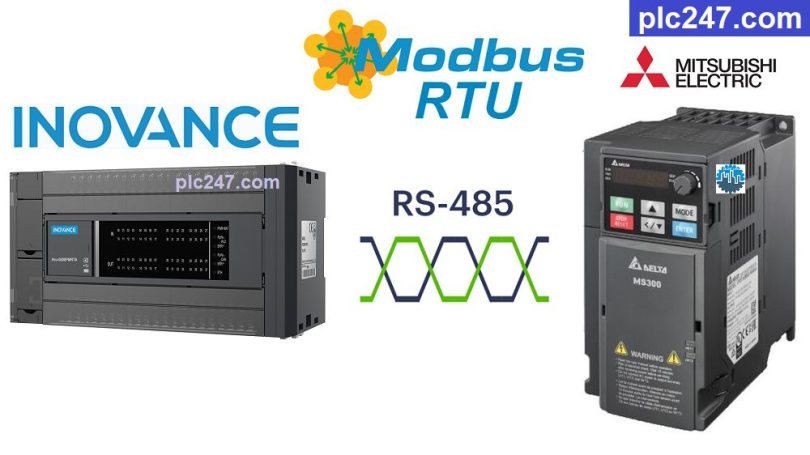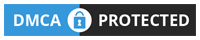# PLC Inovance “Modbus RTU” Delta MS300 TutorialWritten by

Hello friends, in previous posts plc247.com guided you to use PLC Inovance to control some inverters and thermostats through Modbus-RTU, today plc247.com continues to guide you. use it to control Delta inverter.

The selected Delta inverter model is Delta MS300, it has a built-in RS485 port and can be easily controlled by Inovance H3U-3232MT PLC.

#### PLC Inovance & Delta MS300 “Connection Diagram”

+ Below is the RS485 connection diagram between Inovance PLC and Delta MS300 inverter

#### Delta MS300 Modbus Parameters Setting

To be able to control Delta MS300, we need to set the following communication parameters

+ P00.20 = 1 (Frequency Control via RS485)

+ P00.21 = 2 (Motor Control via RS485)

+ P09.00 = 1 (Address Slave = 1)

+ P09.01 = 9.6 (Baudrate = 9600bps)

+ P09.02 = 0

+ P09.03 = 1.0 (Time Com. Err)

+ P09.04: 14 (Modbus-RTU 8/E/1)

##### Delta MS300 “Modbus RTU” Registers Address

+ VFD Control Address = 2000 (hex)

• Set Word 2000 = 18 (decimal): Forward Run
• Set Word 2000 = 34 (decimal): Reverse Run
• Set Word 2000 = 1 (decimal): Stop

+ Frequency Setting Address = 2001 (hex)

+ Output Frequency Address = 2103 (hexa)
Output Current Address = 2104 (hexa)
Output Voltage Address = 2106 (hexa)

#### PLC Inovance “Modbus RTU” Configuration

How have you installed the communication parameters for the Delta MS300 inverter, you also need to install the Inovance PLC so that they can connect to the communication.

### PLC Programming Example

+ Modbus Config & Program

### Project Video Tutorial

======

##### References